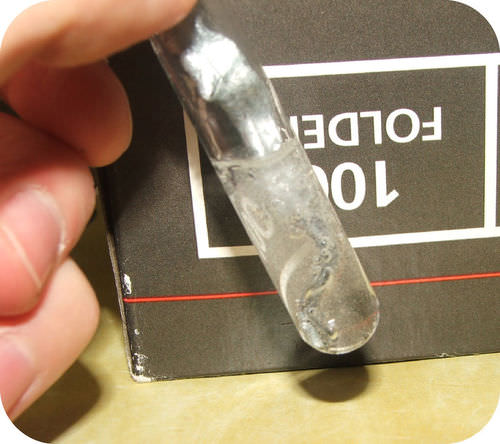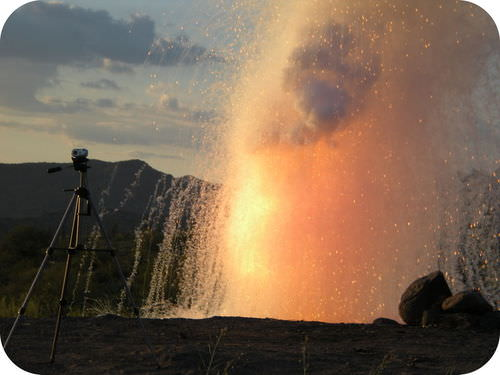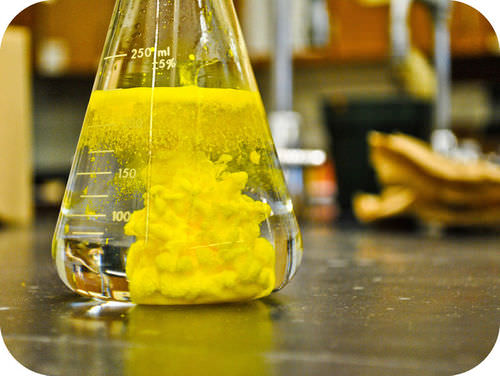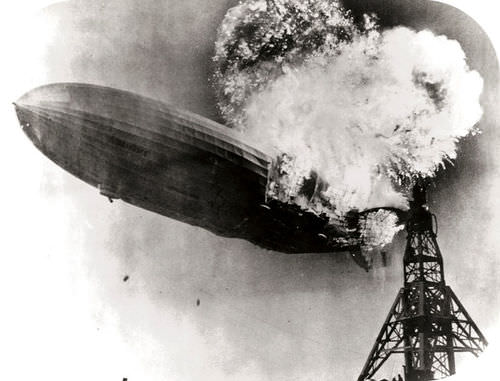# 11.3: Types of Inorganic Reactions

•• Contributed by Allison Soult
• Senior Lecturer (Chemistry) at University of Kentucky

Learning Outcomes

• Classify a reaction as combination, decomposition, single-replacement, double-replacement, or combustion.
• Determine whether a reaction is an oxidation-reduction reaction.
• Predict the products and balance a combustion reaction.

Many chemical reactions can be classified as one of five basic types. Having a thorough understanding of these types of reactions will be useful for predicting the products of an unknown reaction. The five basic types of chemical reactions are combination, decomposition, single-replacement, double-replacement, and combustion. Analyzing the reactants and products of a given reaction will allow you to place it into one of these categories. Some reactions will fit into more than one category. Identifying a reaction as one of these types does not preclude it from also being an oxidation-reduction (redox) reaction. For example, many combination reactions can also be classified as a redox reaction.

## Combination Reactions

A combination reaction, also known as a synthesis reaction, is a reaction in which two or more substances combine to form a single new substance. Combination reactions can also be called synthesis reactions .The general form of a combination reaction is:

$\ce{A} + \ce{B} \rightarrow \ce{AB}$

One example of a combination reaction is two elements combining to form a compound. Solid sodium metal reacts with chlorine gas to product solid sodium chloride.

$2 \ce{Na} \left( s \right) + \ce{Cl_2} \left( g \right) \rightarrow 2 \ce{NaCl} \left( s \right)$

This reaction can also be classified as a redox reaction due to the changes in oxidation states. Sodium goes from a 0 to +1 oxidation state while chlorine goes from a 0 to a $$-1$$ oxidation state.

Notice that in order to write and balance the equation correctly, it is important to remember the seven elements that exist in nature as diatomic molecules ($$\ce{H_2}$$, $$\ce{N_2}$$, $$\ce{O_2}$$, $$\ce{F_2}$$, $$\ce{Cl_2}$$, $$\ce{Br_2}$$, and $$\ce{I_2}$$).

One sort of combination reaction that occurs frequently is the reaction of an element with oxygen to form an oxide. Metals and nonmetals both react readily with oxygen under most conditions. Magnesium reacts rapidly and dramatically when ignited, combining with oxygen from the air to produce a fine powder of magnesium oxide.

$2 \ce{Mg} \left( s \right) + \ce{O_2} \left( g \right) \rightarrow 2 \ce{MgO} \left( s \right)$

## Decomposition Reactions

A decomposition reaction is a reaction in which a compound breaks down into two or more simpler substances. The general form of a decomposition reaction is:

$\ce{AB} \rightarrow \ce{A} + \ce{B}$

Most decomposition reactions require an input of energy in the form of heat, light, or electricity.

Binary compounds are compounds composed of just two elements. The simplest kind of decomposition reaction is when a binary compound decomposes into its elements. Mercury (II) oxide, a red solid, decomposes when heated to produce mercury and oxygen gas.

$2 \ce{HgO} \left( s \right) \rightarrow 2 \ce{Hg} \left( l \right) + \ce{O_2} \left( g \right)$

A reaction is also considered to be a decomposition reaction even when one or more of the products is still a compound. A metal carbonate decomposes into a metal oxide and carbon dioxide gas. For example, calcium carbonate decomposes into calcium oxide and carbon dioxide.

$\ce{CaCO_3} \left( s \right) \rightarrow \ce{CaO} \left( s \right) + \ce{CO_2} \left( g \right)$

Metal hydroxides decompose on heating to yield metal oxides and water. Sodium hydroxide decomposes to produce sodium oxide and water.

$2 \ce{NaOH} \left( s \right) \rightarrow \ce{Na_2O} \left( s \right) + \ce{H_2O} \left( g \right)$

## Single-Replacement Reactions

A single-replacement reaction, also known as single-displacement or substitution reaction, is a reaction in which one element replaces a similar element in a compound. The general form of a single-replacement reaction is:

$\ce{A} + \ce{BC} \rightarrow \ce{AC} + \ce{B}$

In this general reaction, element $$\ce{A}$$ is a metal and replaces element $$\ce{B}$$, also a metal, in the compound. When the element that is doing the replacing is a nonmetal, it must replace another nonmetal in a compound, and the general equation becomes:

$\ce{Y} + \ce{XZ} \rightarrow \ce{XY} + \ce{Z}$

$$\ce{Y}$$ is a nonmetal and replaces the nonmetal $$\ce{Z}$$ in the compound with $$\ce{X}$$.

Magnesium is a more reactive metal than copper. When a strip of magnesium metal is placed in an aqueous solution of copper (II) nitrate, it replaces the copper. The products of the reaction are aqueous magnesium nitrate and solid copper metal.

$\ce{Mg} \left( s \right) + \ce{Cu(NO_3)_2} \left( aq \right) \rightarrow \ce{Mg(NO_3)_2} \left( aq \right) + \ce{Cu} \left( s \right)$

Many metals react easily with acids, and, when they do so, one of the products of the reaction is hydrogen gas. Zinc reacts with hydrochloric acid to produce aqueous zinc chloride and hydrogen (see figure below).

$\ce{Zn} \left( s \right) + 2 \ce{HCl} \left( aq \right) \rightarrow \ce{ZnCl_2} \left( aq \right) + \ce{H_2} \left( g \right)$Figure $$\PageIndex{1}$$: Zinc metal reacts with hydrochloric acid to give off hydrogen gas in a single-replacement reaction.Figure $$\PageIndex{2}$$: Pictured here is about 3 pounds of sodium metal reacting with water. Sodium metal reacts vigorously when dropped into a container of water, giving off hydrogen gas. A large piece of sodium will often generate so much heat that the hydrogen will ignite.

## Double-Replacement Reactions

A double-replacement reaction, also known as double-displacement, is a reaction in which the positive and negative ions of two ionic compounds exchange places to form two new compounds. The general form of a double-replacement reaction is:

$\ce{AB} + \ce{CD} \rightarrow \ce{AD} + \ce{CB}$

In this reaction, $$\ce{A}$$ and $$\ce{C}$$ are positively-charged cations, while $$\ce{B}$$ and $$\ce{D}$$ are negatively-charged anions. Double-replacement reactions generally occur between substances in aqueous solution. In order for a reaction to occur, one of the products is usually a solid precipitate, a gas, or a molecular compound such as water.

A precipitate forms in a double-replacement reaction when the cations from one of the reactants combine with the anions from the other reactant to form an insoluble ionic compound. When aqueous solutions of potassium iodide and lead (II) nitrate are mixed, the following reaction occurs.

$2 \ce{KI} \left( aq \right) + \ce{Pb(NO_3)_2} \left( aq \right) \rightarrow 2 \ce{KNO_3} \left( aq \right) + \ce{PbI_2} \left( s \right)$Figure $$\PageIndex{3}$$: When a few drops of lead (II) nitrate are added to a solution of potassium iodide, a yellow precipitate of lead (II) iodide immediately forms in a double-replacement reaction.

## Combustion Reactions

A combustion reaction is a reaction in which a substance reacts with oxygen gas, releasing energy in the form of light and heat. Combustion reactions must involve $$\ce{O_2}$$ as one reactant. The combustion of hydrogen gas produces water vapor (see figure below).

$2 \ce{H_2} \left( g \right) + \ce{O_2} \left( g \right) + 2 \ce{H_2O} \left( g \right)$

Notice that this reaction also qualifies as a combination reaction.Figure $$\PageIndex{4}$$: The Hindenburg was a hydrogen-filled airship that suffered an accident upon its attempted landing in New Jersey in 1937. The hydrogen immediately combusted in a huge fireball, destroying the airship and killing 36 people. The chemical reaction was a simple one: hydrogen combining with oxygen to produce water.

Many combustion reactions occur with a hydrocarbon, a compound made up solely of carbon and hydrogen. The products of the combustion of hydrocarbons are always carbon dioxide and water. Many hydrocarbons are used as fuel because their combustion releases very large amount of heat energy. Propane $$\left( \ce{C_3H_8} \right)$$ is a gaseous hydrocarbon that is commonly used as the fuel source in gas grills.

$\ce{C_3H_8} \left( g \right) + 5 \ce{O_2} \left( g \right) \rightarrow 3 \ce{CO_2} \left( g \right) + 4 \ce{H_2O} \left( g \right)$

Example $$\PageIndex{1}$$

Ethanol can be used as a fuel source in an alcohol lamp. The formula for ethanol is $$\ce{C_2H_5OH}$$. Write the balanced equation for the combustion of ethanol.

Solution

Step 1: Plan the problem.

Ethanol and oxygen are the reactants. As with a hydrocarbon, the products of the combustion of an alcohol are carbon dioxide and water.

Step 2: Solve.

Write the skeleton equations: $$\ce{C_2H_5OH} \left( l \right) + \ce{O_2} \left( g \right) \rightarrow \ce{CO_2} \left( g \right) + \ce{H_2O} \left( g \right)$$

Balance the equation.

$\ce{C_2H_5OH} \left( l \right) + 3 \ce{O_2} \left( g \right) \rightarrow 2 \ce{CO_2} \left( g \right) + 3 \ce{H_2O} \left( g \right)$